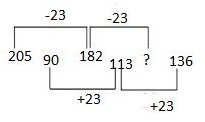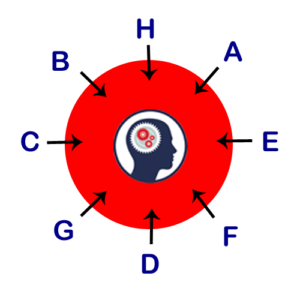# Full Set of Questions Asked in UIIC Assistant Prelims Held on 22nd Sep 2017 (All Slots)

Full Set of Questions Asked in UIIC Assistant Prelims Held on 22nd Sep 2017 (All Slots):

Dear Readers, UIIC Assistant Prelims 2017 examination was completed successfully, here we have given the full set of questions asked in UIIC Assistant Prelims Exam which was held on 22nd Sep 2017. Candidatescan make use of it.

UIIC Assistant Prelims Exam (22nd Sep 2017)

Number Series:

1). 1, 2.1, 3.7, 5.9, 8.8, ?
Difference of Difference 0.5, 0.6, 0.7, 0.8
2). 250, 269, 231, 288, ?, 307
Difference 19×1, 19×2, 19×3, 19×4
3). 109, 107, 112, 102, 119
Difference of Difference 3, 5, 7, 9
4). 16, 8, 8, 12, 24, ?
X0.5, x1, x1.5, x2, x2.5
5). 2, 3, 7, 22, ?
X1+1, x2+1, x3+1, x 4+1

6). 2,   5.8,   9.1,   11.8,   13.8,    ?

Logic :

+3.8…………+3.3………..+2.7………..+2…………+1.2

……-0.5………….-0.6…………-0.7………..-0.8…….

7). 8,   7,   13,   38,   ?,   754

Logic:  ×1 – 1,  ×2 – 1,   ×3 – 1,  ×4 – 1

8). 8, 4, 4, 8, 32, ??

Logic: ×0.5, ×1, ×2, ×4, ×8,

9). 600, 583, 549, 481, ???

Logic: Diff is 17, 34, 68, 136

10). 5,   5,   8,   16,   31,   ?

Logic:

+0…………+3………..+8………..+15…………+24

……+3………….+5…………+7………..+9…….

11). 9, 5, 6, 10.5, 23,?
X0.5+0.5, x1+1, x1.5+1.5, x2+2, x2.5+2.5
12). 189 ,191 ,182 , 210, 145 , ?
Logic: +2(13+1), -9(23+1), +28(33+1), -65(43+1), +126(53+1)
13) 8, 11, 19, 33, 54, ?
Difference +3, +8, +14, +21, +29
Difference of Difference +5, +6, +7, +8
14). 205, 90, 182, 113, ?, 136Answer: 159
15). 1 2 6 21 ?
Logic: ×1 + 1, ×2+2, ×3+3, ×4+4,

Application Sums:

1). The ratio of time taken to complete a piece of work by A and B is 3:5. Together they take 7.5 days to complete the work. Calculate the time taken by A alone to complete the work.

2). A and B invested some amount in a business. After 4 months, C joined them. The difference between the profits of A and C were given. B’s profit had to be calculated.

3). The difference between the marked price and cost price of a certain product is Rs. 60/-. The profit obtained from the sale of that item is Rs. 360/-. If a discount of 20% was given, calculate the Cost price of that item.

4). The perimeter of 2 squares are 64 & 32 metres respectively. Find the perimeter of the third square, whose area is equal to the difference between the areas of first 2 squares?

5). The price of 8 plates and 3 bottles is Rs. 525/-. If the price of 1plate is equal to 1.5 times the price 1 bottle, then find the price of 2 plates and 2 bottles?

6). A boat can row downstream for 13.2 km in 36mins. If the speed of the boat in still water is 20 kmph, then how what distance will the boat travel upstream in 32 minutes?

7). Sum of the Numbers is 12. If the Second Number is reversed, then the number is less than X by 18. Find the numbers?

8). Train A 150m long crosses platform 225m long in 25 sec find time req to cross another train B 225m long with 10m/s in same direction.

9). Mixture Initial ratio of Milk and Water 5:1. 12 lit of mix taken out and 16 lit water added.New Percentage of Water was 30%of mixture find initial mix quantity

10). COST PRICE of an article is Rs.750. It is sold at 20% discount on its Marked price. The sum of Market price and selling price is Rs.1800. Find the SALE PRICE?

11). A 160 ltr mixer contains milk and water in the ratio 3:1.Some quantity of mixture was taken out.Then 29 ltr & 03 ltr of milk and water was added respectively to the mixer.If the quantity of water in the mixer now is 40 ltr Find the amount of mixer taken out.

12). A and B can complete the work in the ratio 3:5 together finish the work in 7 1/2 days how many days A alone done the work?

13). A, B, C, D are invested in the ration of 2: 3: 4 : 9. The total share of A and D is 55000 then what is the total share of C and D?

15). Sum of three numbers is 200. If the Second number is one-third of First and third number is One third of First then find the difference between First and third number?

Floor Puzzle:

1). Eight persons P, Q, R, S, T, U, V and W lives in eight storey building but not necessarily in the same order. Lower most floor is numbered as one and the above floor is numbered as two and so on.
T lives in sixth floor and there is one person lives between T and Q. R lives in even numbered floor immediately above W but does not live in second floor. The number of persons live below W is same as the persons live above P. Three persons live between U and S but U lives above S.

Solution:

8- R
7-W
6-T
5-U
4-Q
3-V
2-P
1-S

2). Eight persons E, F, G, H, I, J, K and L are living in a Eight storey building. Lowest most floor is numbered in 1 and the topmost floor is numbered 8. All the above information is not necessarily in the same order.

E lives in a Even numbered floor but not in second floor. H lives immediately above E. Number of persons below E is same as above G. F lives immediately below I who lives in  a odd numbered floor. J lives one of the floors above K but below L.

Circular Arrangement

1). Eight persons A, B, C, D, E, F, G and H are sitting in circular table facing the center. Two persons are sitting between B and D. E sits second to the right of D. Three persons sit between D and H. A sits immediate right of E. One person sits between F and G. One person sits between A and F.

Solution:2). Eight persons J, K, L, M, N, O, P and Q sitting in a Circular table facing center but not necessarily in the Same order. M sits third to the Right of P. Only one person sits between M and J. L sits second to the left of O. L sits neither immediate neighbor of M nor K. Three person sits between Q and K. Q is not an Immediate of O.

Blood Relation:

R is son of G. G is mother in law of T. T is the son of Q. Q is the grandfather of L. Only one child of Q is married.

Direction:

A is 15m north of D. C is 5m east of D. C is 6m north of E. E is 10m east of B. F is 21m north of B.

Alphanumeric Series:

1). How many such pairs of letters are there in the word NATIVELY which have as many letters between them in the word (both forward and backward) as in the alphabetical order?

2). How many digits are remain in the same position when digits in the number 8132675 arranged in ascending order from left to right?

3). Four of the following five are alike in a certain way. Find which one of the following does not belong to the group?

a) BDEA

b) GIJF

c) LNOK

d) RSTQ

e) WYZV

4). How many such pairs of letters are there in the word ‘IMAGES’ which have as many letters between them in the word as in the alphabetical order?

Syllogism:

1) Statements:

All hills are mountain.

All mountains are peaks.

Conclusions:

1. All hills are peak
2. Some peaks are mountain

2) Statements:

All rats are cat.

Some cats are bat.

Conclusions:

1. Some rats are bat
2. No bat is a rat